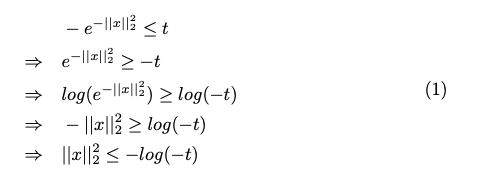# How to solve the optimization problem?

My problem is like the below figure, I try to transform it to the convex problem. Thus, I take the “log” of both sides of the equation and use the bisection algorithm. After programing the Matlab code and run the code, the result is NaN. How do I debug it?

• CVX problem

• Matlab code
r=0.5;
l=1;
u=2;
t=(l+u)/2;
e=1e-8;
while(u-l>e)
cvx_begin
variables x(n)
minimize t
subject to
sum_square((x(n)))+log(t)+ipi<=0
norm(A
x-b,1)<=r
cvx_end
if strfind(cvx_status,‘Solved’)
u=t;
else
l=t;
end
end

How did you get the transformed problem? That doesn’t look correct to me.

The original objective function is wrong. The new equation is below.

My transform processIs ||x|_2^2 + log(-t) \le 0 a convex constraint? No. Therefore I believe you have attempted quasi-convex alchemy.

Hi Mark,

Do I transform it to the right convex problem?
Why when I run the code the cvx_optval is NaN?

Best

I was trying to say I don’t believe what you have done is valid.

I don’t know what code you are running. if you don’t use the quiet option, the solver and CVX output should be displayed, which should explain why the results are NaN. But that presupposes CVX accepted your problem and did not produce an error message before calling the solver. A <= inequality with -log on the RHS will be rejected by CVX.

I know that the CVX will reject it, so I revise the transform process to the new constraint ||x||_2^2 \leq log(t)+i*pi

I have post the final transform problem at the beginning.

I use the transform problem to program it .

The below is my Matlab code

tr=0.5;
l=1;
u=2;
t=(l+u)/2;
e=1e-8;
while(u-l>e)
cvx_begin
variables x(n)
minimize t
subject to
sum_square((x(n)))+log(t)+i*pi<=0
norm(A*x-b,1)<=r
cvx_end
if strfind(cvx_status,‘Solved’)
u=t;
else
l=t;
end
end


What does this have to do with the problem you’re trying to solve? What is i*pi supposed to do?

I don’t believe you are following the instructions for Bisection in section 4.2.5 of https://web.stanford.edu/~boyd/cvxbook/bv_cvxbook.pdf .Nor, as far, as I know,is it possible to do so on your problem.